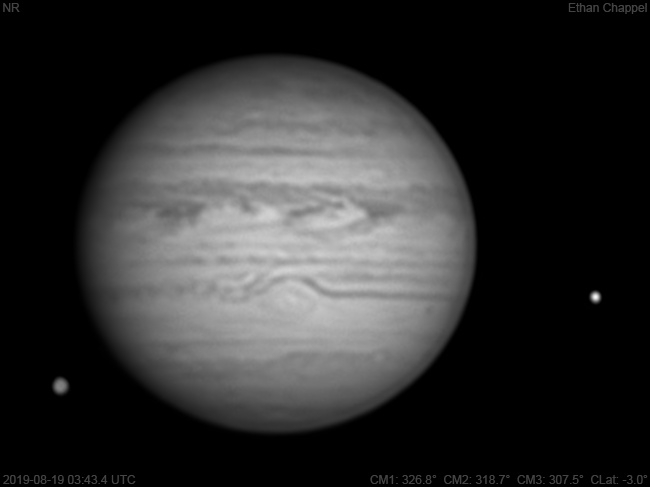# Jupiter 2019-08-19 03:43 UTC

CM1: 326.80°

CM2: 318.70°

CM3: 307.50°

CLat: -3.00°

Description

The best conditions for imaging I've had with the C8 so far this summer, although it got a little worse by the time I got to recording methane data.

Notes:

• White Oval Z is bright on its north side.
• The Equatorial Zone has lots of activity on it's boundary with the North Equatorial Belt.
• The Great Red spot is front and center.
• More than half of the long and faint STB Spectre has passed the GRS.
• Io is approaching occultation as Ganymede emerges from behind Jupiter.

Equipment

ZWO ASI290MM

Celestron EdgeHD 8

Logs
```FireCapture v2.6  Settings
------------------------------------
Observer=Ethan Chappel
Camera=ZWO ASI290MM
Filter=IR
Profile=Jupiter
Diameter=40.48"
Magnitude=-2.30
CMI=328.0° CMII=320.0° CMIII=308.7°  (during mid of capture)
FocalLength=3700mm (F/13)
Resolution=0.16"
Filename=2019-08-19-0344_7-EC-IR-Jup.ser
Date=2019_08_19
Start=03_43_31.080
Mid=03_44_46.081
End=03_46_01.082
Start(UT)=03_43_31.080
Mid(UT)=03_44_46.081
End(UT)=03_46_01.082
Duration=150.002s
Date_format=yyyy_MM_dd
Time_format=HH_mm_ss
LT=UT -6h
Frames captured=9997
File type=SER
Binning=no
Bit depth=8bit
Debayer=no
ROI=576x312
ROI(Offset)=8x8
FPS (avg.)=66
Shutter=15.00ms
Gain=300 (50%)
HighSpeed=off
SoftwareGain=10 (off)
AutoExposure=off
AutoGain=off
AutoHisto=75 (off)
USBTraffic=40 (off)
Gamma=50
FPS=100 (off)
Brightness=1 (off)
Histogramm(min)=0
Histogramm(max)=130
Histogramm=50%
Noise(avg.deviation)=0.42
AutoAlign=false
PreFilter=none
Limit=150 Seconds
Sensor temperature=36.8°C
FireCapture v2.6  Settings
------------------------------------
Observer=Ethan Chappel
Camera=ZWO ASI290MM
Filter=IR
Profile=Jupiter
Diameter=40.48"
Magnitude=-2.30
CMI=326.3° CMII=318.3° CMIII=307.1°  (during mid of capture)
FocalLength=3700mm (F/13)
Resolution=0.16"
Filename=2019-08-19-0342_0-EC-IR-Jup.ser
Date=2019_08_19
Start=03_40_45.569
Mid=03_42_00.570
End=03_43_15.571
Start(UT)=03_40_45.569
Mid(UT)=03_42_00.570
End(UT)=03_43_15.571
Duration=150.002s
Date_format=yyyy_MM_dd
Time_format=HH_mm_ss
LT=UT -6h
Frames captured=9998
File type=SER
Binning=no
Bit depth=8bit
Debayer=no
ROI=576x312
ROI(Offset)=8x8
FPS (avg.)=66
Shutter=15.00ms
Gain=300 (50%)
HighSpeed=off
SoftwareGain=10 (off)
AutoExposure=off
AutoGain=off
AutoHisto=75 (off)
USBTraffic=40 (off)
Gamma=50
FPS=100 (off)
Brightness=1 (off)
Histogramm(min)=0
Histogramm(max)=129
Histogramm=50%
Noise(avg.deviation)=0.42
AutoAlign=false
PreFilter=none
Limit=150 Seconds
Sensor temperature=36.7°C
```